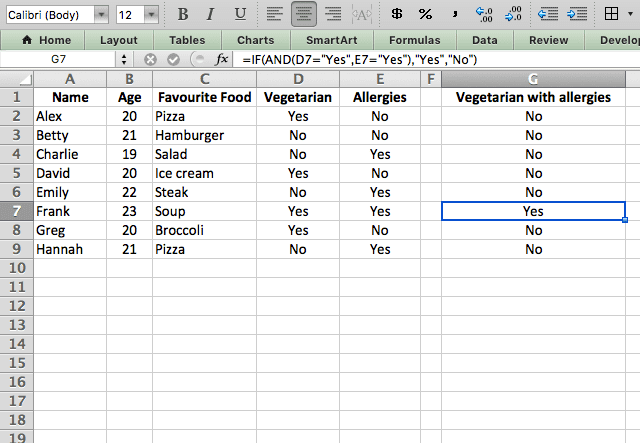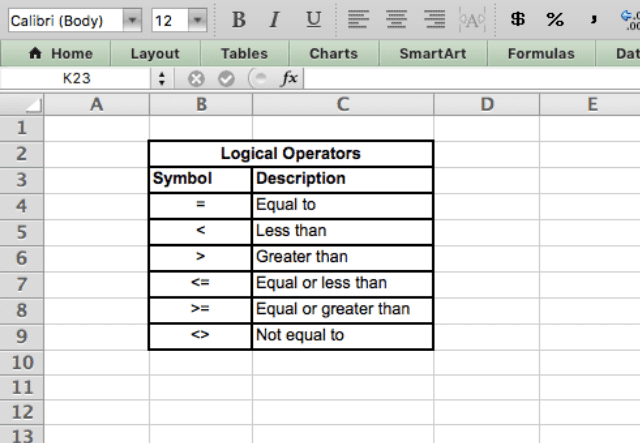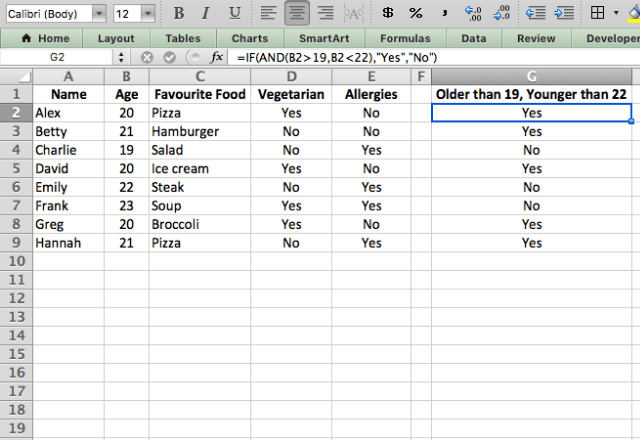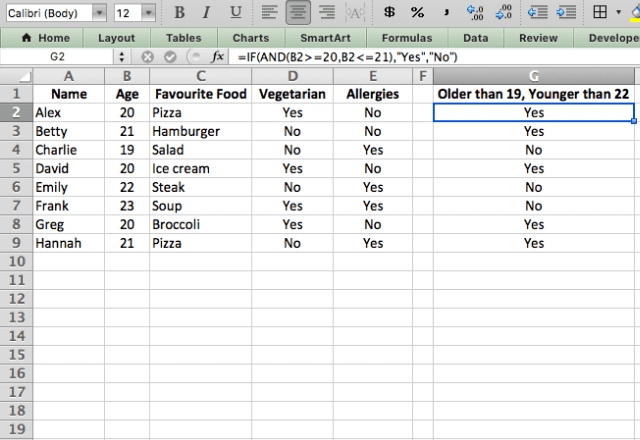# How to use the IF AND function combination in ExcelThe IF function in Excel is extremely useful: it generates different outcomes depending on whether the input meets a certain criteria. But what if you want the input to meet multiple criteria instead of just one? That’s where the AND function comes in. When combined, the IF AND Excel functions create an even more powerful tool!

### Syntax

I’ll start off by showing the syntax of a typical IF function:

=IF(logical_test, value_if_true, [value_if_false])
• logical_expression – an expression or reference to a cell containing an expression that has a logical value such as TRUE or FALSE. The AND function is used here to test multiple expressions
• value_if_true – the value that the function returns if the logical_expression is TRUE. This can be a number, text, or even another function that returns a value. You can also embed another IF statement in here: this is known as a nested IF
• value_if_false – the value that the IF function returns if the logical_expression is FALSE. Similar to the value_if_true, this can be a number, text or another function that returns a value. It can also be a nested IF. Note that this is an optional input and if you leave it out you will get a blank value when the expression is FALSE

Now for the AND function:

=AND(logical1, [logical2], …)
• logical1, logical2, etc. are logical expressions. The function will generate a TRUE result only if all the logical expressions are true

Finally, when you put them together you get:

=IF(AND(logical1, [logical2], …), value_if_true, [value_if_false])
• When combined, the AND function takes the place of the logical_expression parameter in the IF function
• All the logical expressions in the AND function must be TRUE in order to get the value_if_true

### How to use the IF AND combination

I’ll show a couple examples now in order to fully explain the functions. In this example, I have a list of people and their dietary information. I want to write a function that will tell me whether each person is a vegetarian and has allergies.

I can do this with the formula =IF(AND(D2=”Yes”,E2=”Yes”),”Yes”,”No”).The logical expression here is AND(D2=”Yes”,E2=”Yes”). This expression only evaluates as TRUE when both the values in column D (vegetarian) and E (allergies) are Yes. If either of them are No, then the expression is FALSE.

Note that you can use any of the logical operators in your logical expression. The list below shows the options you have.#### Number between two values

Another way you can use the IF AND Excel function combination is when you want to find a number that’s between two numbers.

For example, if I want to see who is older than 19 but younger than 22, I can use the function =IF(AND(B2>19,B2<22),"Yes","No").Here I’ve used two more of the logical operators shown previously (> and <). Using the AND function allows me to quickly set a range for each value by defining the upper and lower limits.

I could also use the >= and <= operators to get the same result, by typing =IF(AND(B2>=20,B2<=21),"Yes","No").### Tips for using the IF AND function combination in Excel

• The IF AND combination is a much cleaner alternative to nested IF statements. Sometimes nested IF’s are necessary, but if you can use IF and AND instead, do it!
• All logical expressions within the AND function must be true, otherwise it will return FALSE
• If you want to get a TRUE result if any of your logical expressions is true, you can use the OR function instead of AND